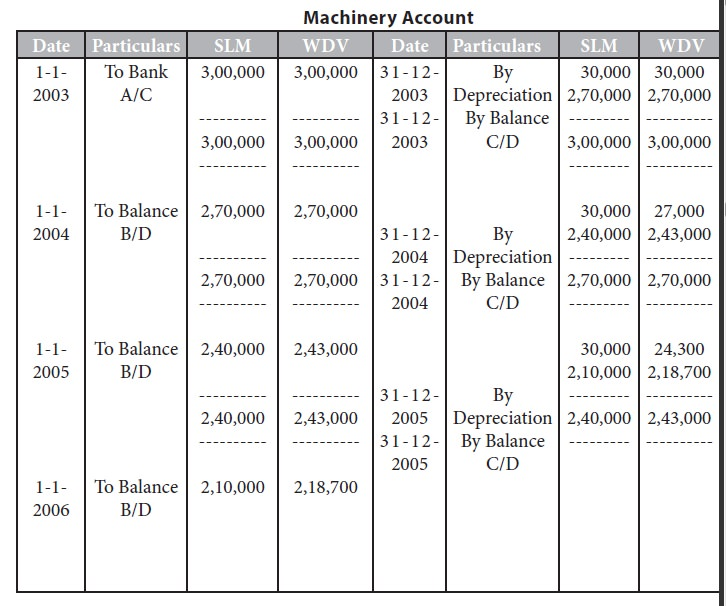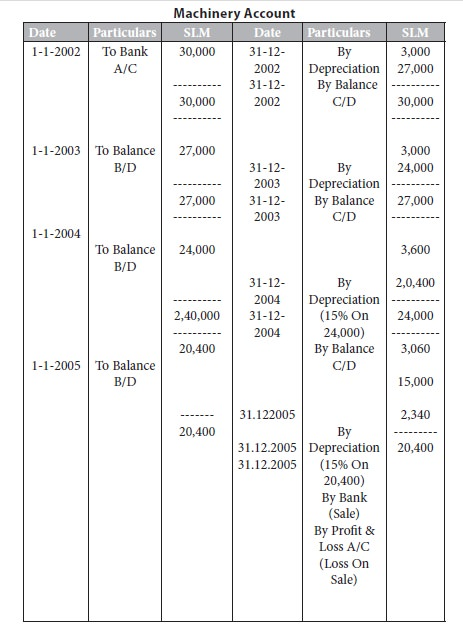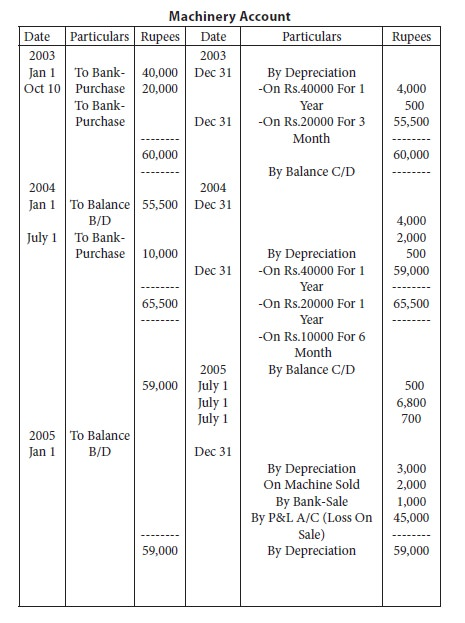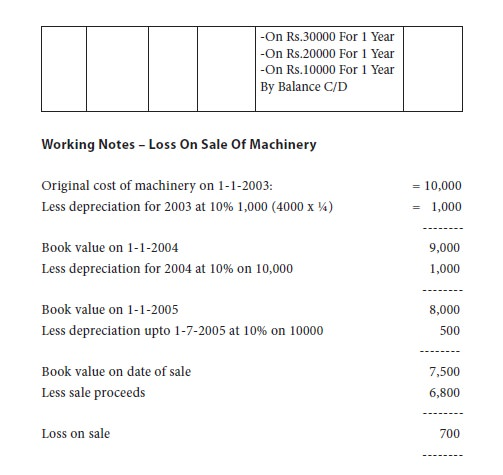Home | ARTS | Accounting For Managers | Methods Of Depreciation - Straight line method, Diminishing balance method and Annuity method are discussed

# Methods Of Depreciation - Straight line method, Diminishing balance method and Annuity method are discussed

Posted On :  26.01.2018 06:55 am

The amount of depreciation of a fixed asset is determined taking into account the following three factors: its original cost, its recoverable cost at the time it is retired from service and the length of its life.

Out of these three factors the only factor which is accurately known is the original cost of the asset. The other two factors cannot be accurately determined until the asset is retired. They must be estimated at the time the asset is placed in service. The excess of cost over the estimated residual value is the amount that is to be recorded as depreciation expense during the assets’ life-time. There are no hard and fast rules for estimating either the period of usefulness of an asset or its residual value at the end of such period. Hence these two factors, which are inter-related are affected to a considerable extent by management policies.

Let the reader consider the following example: a machine is purchased for rs.1,00,000 with an estimated life of five years and estimated residual value of rs.10,000. The objective of depreciation accounting is to charge this net cost of rs.90,000 (original cost – residual value) as an expense over the 5 year period. How much should be charged as an expense each year? To give an answer to this question a 100 number of methods of depreciation are available. In this lesson three such methods viz.

1.Straight line method,

2.Diminishing balance method and

3.Annuity method are discussed.

Straight Line Method Of Depreciation

This method which is also known as ‘fixed installment system’, provides for equal amount of depreciation every year. Under this method, the cost of acquisition plus the installation charges, minus the scrap value, is spread over the estimated life of the asset to arrive at the annual charge. In other words, this method writes off a fixed percentage, say 20%, of the original cost of the asset every year in such a way that the asset is reduced to nil or scrap value at the end of its life.

Evaluation:

The chief merit of this method is that it is easy to calculate depreciation, and hence, it is simple. Depreciation charge is constant from year to year, regardless of the extent of use of the asset. This method can be employed in the case of assets like furniture and fixtures, short leases, etc., which involve little capital outlay, or which have no residual value. This method is criticized on the ground that the depreciation charge remaining the same every year, cost of repairs and maintenance would be increasing as the asset becomes older. With the efficiency of the asset declining, it is unfair to charge the same amount of depreciation every year.

Diminishing Balance Method

This method which is also known as the, `reducing installment system’, or `written down value method’, applies depreciation as a fixed percentage to the balance of the net cost of the asset not yet allocated at the end of the previous accounting period. The percentage of depreciation is so fixed that, theoretically, the balance of the unallocated cost at the end of the estimated useful life of the asset should be equal to the estimated residual value.

Evaluation:

Unlike the fixed installment system, depreciation under this method is not fixed, but gradually decreasing. As such, in the initial periods, the amount will be much higher, but negligible in the later period of the asset. Thus, this method tends to offset the amount of depreciation on the one hand and repairs and maintenance on the other. This method is also simple, although the calculation of depreciation is a bit complicated. Further, as and when additions are made to the asset, fresh calculations do not become necessary. This method is best suited to assets such as plant and machinery which have a long life.

Entries Required:

The entry to be made on writing off depreciation under any method is:

Depreciation a/c ….. Dr

To asset a/c

The depreciation account goes to the debit of the profit and loss account. The entry for this is:

Profit and loss a/c … dr

To depreciation a/c

The asset appears at its reduced value in the balance sheet.

llustration 1:

On 1-1-2003, machinery was purchased for rs.3,00,000. Depreciation at the rate of 10% has to be written off. Write up the machinery account for three years under:

1.         Straight line method (SLM) and

2.         Written down value method (WDV)Solution:

Machinery Account

From the above illustration it can be seen that under SLM method each year depreciation is calculated at 10% on original cost of asset i.e. On rs.3,00,000, while under WDV method each year depreciation is calculated at 10% on the written down value i.e. For e.g. In the 2nd year depreciation is calculated at 10% on rs.2,70,000 and so on.

Illustration 2:

On 1-1-2002, machinery was purchased for rs.30,000. Depreciation at the rate of 10% on original cost was written off during the first two years. For the next two years 15% was written off the diminishing balance of the amount. The machinery was sold for rs.15,000. Write up the machinery account for four years and close the same.Illustration 3:

A company, whose accounting year is the calendar year, purchased machinery on 1-1-2003 for rs.40,000. It purchased further machinery on 1-10-2003 for rs.20,000 and on 1st july 2005 for rs.10,000. On 1-7-2006, one-fourth of the machinery installed on 1-1-2003 became obsolete and was sold for rs.6,800. Show the machinery account for all the 3 years under fixed installment system. Depreciation is to be provided at 10%p.a.Tags : Accounting For Managers - Revenue Recognition
Last 30 days 2168 views